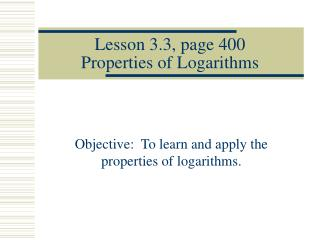DownloadDownload PresentationLesson 3.3, page 400 Properties of Logarithms

# Lesson 3.3, page 400 Properties of Logarithms

Download Presentation## Lesson 3.3, page 400 Properties of Logarithms

- - - - - - - - - - - - - - - - - - - - - - - - - - - E N D - - - - - - - - - - - - - - - - - - - - - - - - - - -
##### Presentation Transcript

1. Lesson 3.3, page 400Properties of Logarithms Objective: To learn and apply the properties of logarithms.

2. Real-World Connection • Logarithms are used in applications involving sound intensity &decibel level.

3. Think about this… • If a logarithm is the inverse of an exponential, what do you think we can surmise about the properties of logarithms? • They should be the inverse of the properties of exponents! For example, if we add exponents when we multiply in the same base, what would we do to logs when they are being multiplied?

4. PRODUCT RULE, page 400 • Product Property: logb(MN) = logbM + logbN The logarithm of a product is the sum of the logarithms of the factors. • Ex)logbx3 + logby =

5. See Example 1, pg. 401 • Express as a single logarithm:

6. Check Point 1 • Use the product rule to expand each logarithmic expression: • A) log6(7 11) B) log(100x)

7. QUOTIENT RULE, page 401 • Quotient Property logb(M/N) = logbM – logbN The logarithm of a quotient is the logarithm of the numerator minus the logarithm of the denominator. Ex) log2w - log216 =

8. See Example 2, page 402. • Express as a difference of logarithms.

9. Check Point 2 • Use the quotient rule to expand each logarithmic expression:

10. POWER RULE, page 402 • Power Property: logbMp = p logbM The logarithm of a power of M is the exponent times the logarithm of M. • Ex) log2x3 =

11. Express as a product. See Example 3, page 403.

12. Check Point 3 • Use the power rule to expand each logarithmic expression:

13. Extra Practice • Express as a product.

14. Expanding Logarithmic Expressions(See blue box on page 403.) • Use properties of logarithms to change one logarithm into a sum or difference of others. • Example

15. See Example 4, page 404 • Check Point 4: Use log properties to expand each expression as much as possible.

16. Expanding Logs – Express as a sum or difference.

17. log27b log(y/3)2 c) log7a3b4 More Practice Expanding

18. Condensing Logarithmic Expressions(See blue box on page 404.) • We can also use the properties of logarithms to condense expressions or “write as a single logarithm”. • See Example 5, page 404.

19. Let’s reverse things. • Express as a single logarithm.

20. Pencils down. Watch and listen. • Express as a single logarithm. • Solution:

21. Check Point 5 • Write as a single logarithm.

22. Check Point 6Write as a single logarithm.

23. Check Point 6Write as a single logarithm.

24. More Practice • d) Write 3log2 + log 4 – log 16 as a single logarithm. • e) Can you write 3log29 – log69 as a single logarithm?

25. Review of Properties(from Lesson 3.2) • The Logarithm of a Base to a Power For any base a and any real number x, loga a x = x. (The logarithm, base a, of a to a power is the power.) • A Base to a Logarithmic Power For any base a and any positive real number x, (The number a raised to the power loga x is x.)

26. Simplify. a) loga a 6 b) ln e 8 Examples

27. Simplify. • A) • B)

28. Change of Base Formula • The 2 bases we are most able to calculate logarithms for are base 10 and base e. These are the only bases that our calculators have buttons for. • For ease of computing a logarithm, we may want to switch from one base to another using the formula

29. See Examples 7 & 8, page 406-7. • Check Point 7: Use common logs to evaluate log7 2506. • Check Point 8: Use natural logs to evaluate log7 2506.

30. Summary of Properties of Logarithms

31. Summary of Properties of Logarithms (cont.)